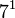# Groups of order 189

## Contents

See pages on algebraic structures of order 189| See pages on groups of a particular order

## Statistics at a glance

The number 189 has prime factorization$189 = 3^3 \cdot 7$. There are only two prime factors of this number. Order has only two prime factors implies solvable (by Burnside's$p^aq^b$-theorem) and hence all groups of this order are solvable groups (specifically, finite solvable groups). Another way of putting this is that the order is a solvability-forcing number. In particular, there is no simple non-abelian group of this order.

Quantity Value Explanation
Total number of groups 13
Number of abelian groups 3 (number of abelian groups of order$3^3$) times (number of abelian groups of order$7^1$) = (number of unordered integer partitions of 3) times (number of unordered integer partitions of 1) =$3 \times 1 = 3$. See classification of finite abelian groups and structure theorem for finitely generated abelian groups.
Number of nilpotent groups 5 (number of groups of order 27) times (number of groups of order 7) =$5 \times 1 = 5$. See number of nilpotent groups equals product of number of groups of order each maximal prime power divisor, which in turn follows from equivalence of definitions of finite nilpotent group.
Number of solvable groups 13 There are only two prime factors of this number. Order has only two prime factors implies solvable (by Burnside's$p^aq^b$-theorem) and hence all groups of this order are solvable groups (specifically, finite solvable groups). Another way of putting this is that the order is a solvability-forcing number. In particular, there is no simple non-abelian group of this order.
Number of simple groups 0 Follows from all groups of this order being solvable.

## GAP implementation

The order 189 is part of GAP's SmallGroup library. Hence, any group of order 189 can be constructed using the SmallGroup function by specifying its group ID. Also, IdGroup is available, so the group ID of any group of this order can be queried.

Further, the collection of all groups of order 189 can be accessed as a list using GAP's AllSmallGroups function.

Here is GAP's summary information about how it stores groups of this order, accessed using GAP's SmallGroupsInformation function:

gap> SmallGroupsInformation(189);

There are 13 groups of order 189.
They are sorted by their Frattini factors.
1 has Frattini factor [ 21, 1 ].
2 has Frattini factor [ 21, 2 ].
3 - 8 have Frattini factor [ 63, 3 ].
9 - 11 have Frattini factor [ 63, 4 ].
12 - 13 have trivial Frattini subgroup.

For the selection functions the values of the following attributes
are precomputed and stored:
IsAbelian, IsNilpotentGroup, IsSupersolvableGroup, IsSolvableGroup,
LGLength, FrattinifactorSize and FrattinifactorId.

This size belongs to layer 2 of the SmallGroups library.
IdSmallGroup is available for this size.